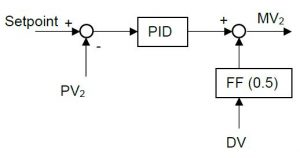## How to design a feedforward controller

A feedforward controller should be designed on the basis of a model having the following form:

$$PV = \frac{K_1}{T_{p,2} s + 1} e^{-T_{DT,1} T_s} DV + \frac{K_2}{T_{p,2} s + 1} e^{-T_{DT,2} T_s} MV$$
Where:
$$PV =$$ Process Variable
$$T_p =$$ Process Time Constant
$$T_{dt} =$$ Process Delay
$$s =$$ LaPlace variable
$$DV =$$ Disturbance Variable.
$$MV =$$ Manipulated Variable.

This model can easily be identified, using the PID-Tuner.

Once the model is identified the feedforward gain can be calculated on the basis of the assumption that the dynamics can be ignored, and that the effect of DV on PV is compensated exactly. Then the gain can be calculated as follows:

$$FF = \frac{K_1}{K_2}$$

## How to implement a feedforward controller

To implement a feedforward controller, the figure as shown below should be used as a reference.Feedforward design